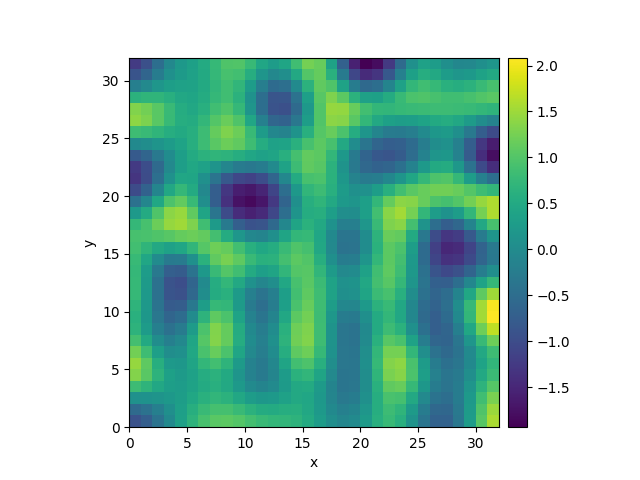# 2.23. Kuramoto-Sivashinsky - Compiled methods

This example implements a scalar PDE using a custom class with a numba-compiled method for accelerated calculations. We here consider the Kuramoto–Sivashinsky equation, which for instance describes the dynamics of flame fronts:

$\partial_t u = -\frac12 |\nabla u|^2 - \nabla^2 u - \nabla^4 u$0%|          | 0/10.0 [00:00<?, ?it/s]
Initializing:   0%|          | 0/10.0 [00:00<?, ?it/s]
0%|          | 0/10.0 [00:10<?, ?it/s]
0%|          | 0.01/10.0 [00:11<3:15:22, 1173.45s/it]
0%|          | 0.02/10.0 [00:11<1:37:35, 586.74s/it]
2%|1         | 0.18/10.0 [00:11<10:40, 65.20s/it]
68%|######7   | 6.75/10.0 [00:11<00:05,  1.74s/it]
68%|######7   | 6.75/10.0 [00:11<00:05,  1.74s/it]
100%|##########| 10.0/10.0 [00:11<00:00,  1.18s/it]
100%|##########| 10.0/10.0 [00:11<00:00,  1.18s/it]


import numba as nb

from pde import PDEBase, ScalarField, UnitGrid

class KuramotoSivashinskyPDE(PDEBase):
"""Implementation of the normalized Kuramoto–Sivashinsky equation"""

def __init__(self, bc="auto_periodic_neumann"):
super().__init__()
self.bc = bc

def evolution_rate(self, state, t=0):
"""implement the python version of the evolution equation"""
state_lap = state.laplace(bc=self.bc)
state_lap2 = state_lap.laplace(bc=self.bc)
return -state_grad_sq / 2 - state_lap - state_lap2

def _make_pde_rhs_numba(self, state):
"""nunmba-compiled implementation of the PDE"""
laplace = state.grid.make_operator("laplace", bc=self.bc)

@nb.jit
def pde_rhs(data, t):
return -0.5 * gradient_squared(data) - laplace(data + laplace(data))

return pde_rhs

grid = UnitGrid([32, 32])  # generate grid
state = ScalarField.random_uniform(grid)  # generate initial condition

eq = KuramotoSivashinskyPDE()  # define the pde
result = eq.solve(state, t_range=10, dt=0.01)
result.plot()


Total running time of the script: ( 0 minutes 11.930 seconds)